## 1. 不需要声明变量

在c++中，变量需要先声明，然后再赋值、使用。

在Python中，变量不需要声明，直接赋值，然后Python会根据值的类型来确定变量的类型。

如：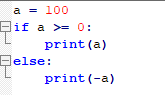## 2. 代码块不使用{ }，在代码结尾不需要“；”

在c++中，代码总是在{ }里面的，如：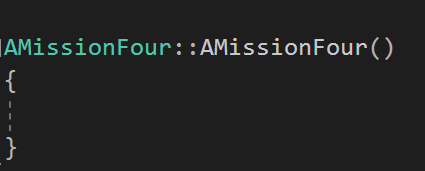但在Python，不需要使用{ }来封装代码块，而是用严格的缩进机制，如：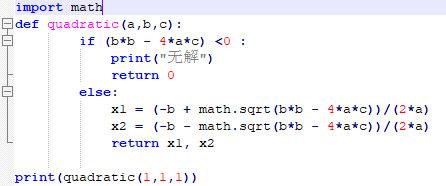注意if else里面的内容，它们是对齐的，这意味着它们属于同一块代码块（当然，if 下面是一个代码块，else下面的是另一个代码块。）

代码块开始前，要加“ ：”，如上图的 if (b*b - 4*a*c) <0 :

if和else也是对齐的，故它们是在同一个代码块中。

注意：“；”也不需要了

## 3.  函数也不需要声明，只需在函数名前加“def”来识别

在c++里，函数需要先在头文件中声明，然后在cpp文件中写函数的本体。

而在Python里，没有头文件，函数写法如下图：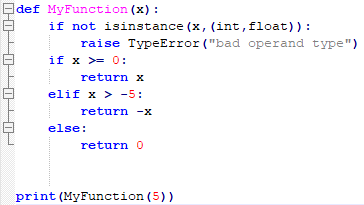要用def来识别函数本体，然后直接调用即可，如图中的print(MyFunction(5))。

注意，最好一开始判断一下变量类型是否是本函数使用的类型。

## 4. 代码量少

Python的代码量相对较少，可以一句代码把c++的一段代码写完，如下图：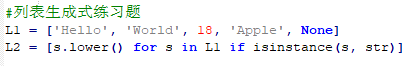L1是一个数组，但里面的数值不一定都是同一个类型。

L2的意思是把L1的字符串全部改为小写，形成新数组。

L2为['hello', 'world', 'apple']。

L2的那句代码称为生成式，里面有一个for循环和一个if判断语句。

## 5. 数组类型

list：普通数组

classmates = ['Michael', 'Bob', 'Tracy']

tuple：一旦初始化就不能修改

classmates = ('Michael', 'Bob', 'Tracy')

dict:与c++的map类似

d = {'Michael': 95, 'Bob': 75, 'Tracy': 85}

set:set和dict类似，也是一组key的集合，但不存储value。

s = set([1, 2, 3])

## 6. global和nonlocal

global是全局变量的关键词；

nonlocal是外层变量的关键词。

全局变量没什么好说的，调用前需要先申明一下，如：（不声明的话，局部变量会覆盖掉全局变量）

#整数部分
intNum = 0
#记录有几位小数
n = 0

def str2float(t):
global intNum
global n

def char2num(s):
DIGITS = {'0': 0, '1': 1, '2': 2, '3': 3, '4': 4, '5': 5, '6': 6, '7': 7, '8': 8, '9': 9}
if s == ".":
return s
else:
return DIGITS[s]
def fn(x,y):
global intNum
global n
if y == "." :
intNum += x
return x
elif intNum > 0 :
n += 1
return x * 10 + y
else :
return x * 10 + y
x = reduce(fn, map(char2num,t))
x = x / (10 ** n)
#算完之后，全局变量初始化
n = 0
intNum = 0
return x

nonlocal用法如下：

#闭包练习题
def createCounter():
n = 0
def counter():
nonlocal n
n += 1
return n
return counter

用nonlocal可以避免污染全局。

在python中，以下写法是错误的：

def createCounter():
n = 0
def counter():
#nonlocal n
n += 1
return n
return counter

编辑器会提示，变量在定义前调用了。这样写在c++中没问题，但在python却成为问题了。

解决办法是用global或者nonlocal。

PS：Python中，可以在函数里面定义函数！

## 7. lambda匿名函数

python缩减代码量的一个手段：lambda

def is_odd(n):
return n % 2 == 1

L = list(filter(is_odd, range(1, 20)))

#使用匿名函数后
L = list(filter(lambda x : x % 2 == 1, range(1, 20)))

上述代码中，两个L是相同的，lambda冒号前面的x表示函数参数。

对比可以发现，lambda代替了函数is_odd(n)。

PS：filter是高阶函数：filter()把传入的函数依次作用于每个元素，然后根据返回值是True还是False决定保留还是丢弃该元素。

## 8. 高阶函数

上面介绍了一种高阶函数filter，接下来介绍map和reduce

map(): 它把传入的函数依次作用于每个元素，依次返回处理后的结果，如：

L = list(map(lambda x : x % 2 == 1, range(1, 20)))

L = [True, False, True, False, True, False, True, False, True, False, True, False, True, False, True, False, True, False, True]

reduce(): 把一个函数作用在一个序列[x1, x2, x3, ...]上，这个函数必须接收两个参数，reduce把结果继续和序列的下一个元素做累积计算，

reduce(f, [x1, x2, x3, x4]) = f(f(f(x1, x2), x3), x4)

如：

L = reduce(lambda x,y :x+y, [1, 3, 5, 7, 9])

L = 25 (相当于1+3+5+7+9)

PS:使用reduce前需要from functools import reduce